trustle > EngliSea > Corona Safe Schooling > mathematics > Quick Math Index > 25 Exponent, root and logarithm

Exponentiation is repeated multiplication. Root is the inverse operation of exponentiation. Logarithm is a new inverse operation of exponentiation.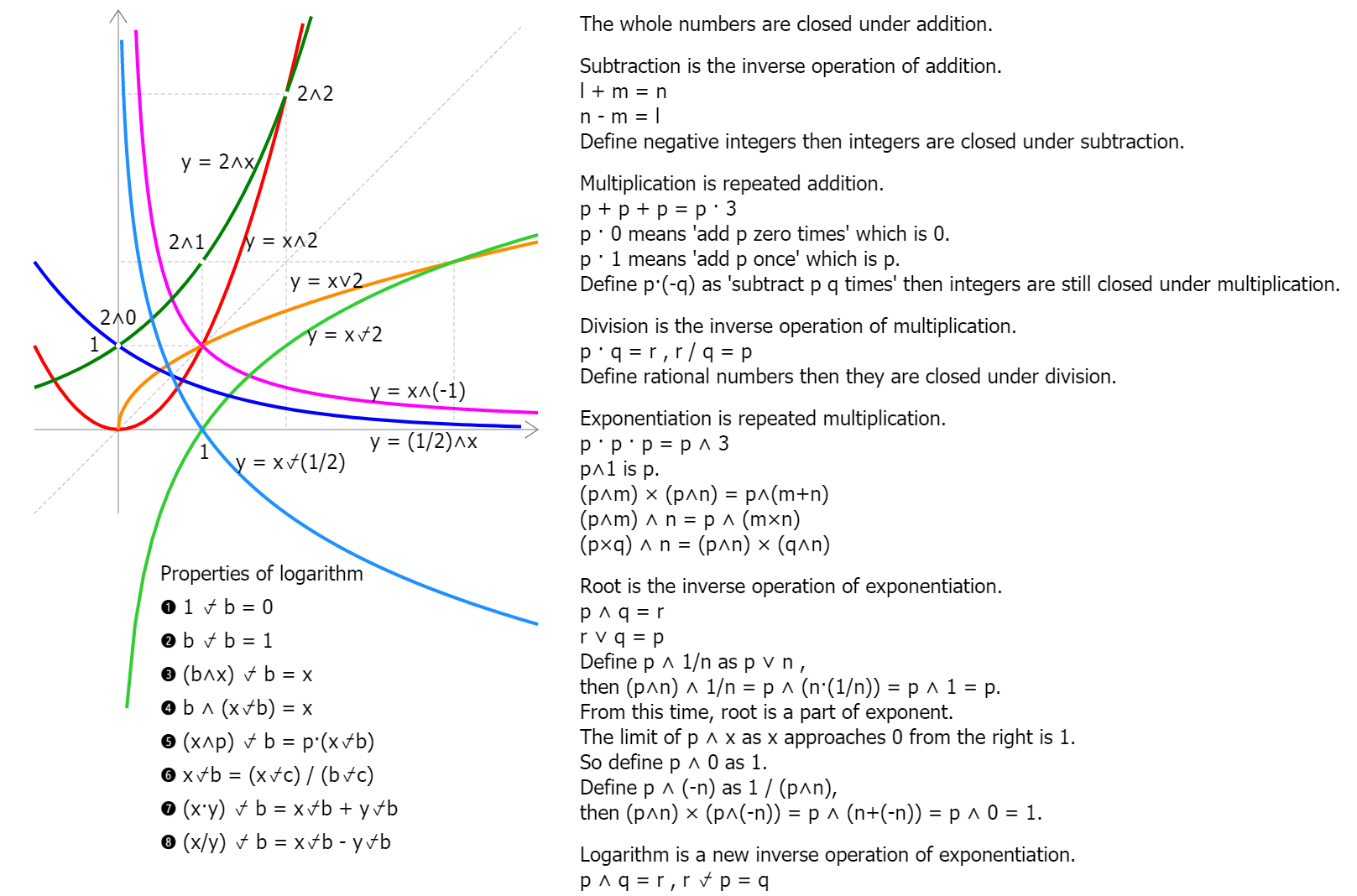Expansion of Numbers and Operations
9550

The whole numbers are closed under addition.

➋ Subtraction is the inverse operation of addition.
l + m = n
n - m = l
Define negative integers then integers are closed under subtraction.

p + p + p = p·3
p ...

➊ 1⍻b = 0
The power that the base must be raised to in order to yield 1 is the 0th.

➋ b⍻b = 1
The power that the base must be raised to in order to yield the base itself is the 1st.

➌ (b˄x)⍻b = x
The power that the base must be raised to in order to yie ...05 Exponents In Algebra
Algebra Basics: Exponents In Algebra - Math Antics
3799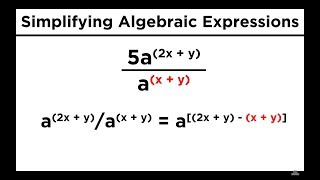08 Simplifying Expressions With Roots and Exponents
Simplifying Expressions With Roots and Exponents
3801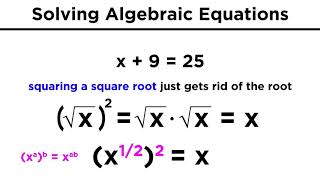09 Solving Algebraic Equations With Roots and Exponents
Solving Algebraic Equations With Roots and Exponents
3802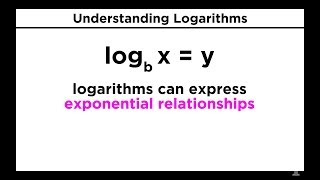41 Logarithms Part 1
When we say log base b of x equals y, we are saying b to the y equals x. With logs, the base of the log raised to the power of what's on the other side of the equal sign will equal the number that the log is operating on.
3638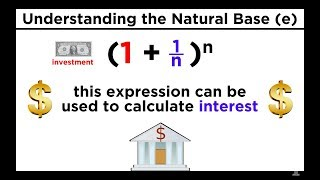42 Logarithms Part 2
Base Ten Logs, Natural Logs, and the Change-Of-Base Property
3639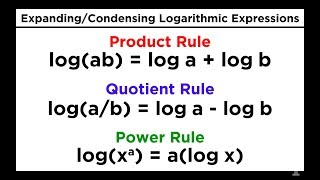43 Logarithms Part 3
Logarithms Part 3: Properties of Logs, Expanding Logarithmic Expressions
3640Change of base
Log base b of n. What power should I put in the exponent of b so b to that power will give me n.
3798exponential function
You can expect the difference between one x value at any point to be the same as any other.
3625Exponentiation
b˄n is called "b raised to the n-th power", "b raised to the power of n", "the n-th power of b", "b to the n-th", or most briefly as "b to the n"
9671

Interest
Simple And Compound Interest And Spreadsheet Intro
9774Logarithm
"the log, base b, of x" "To what power must b be raised, in order to yield x?"
9638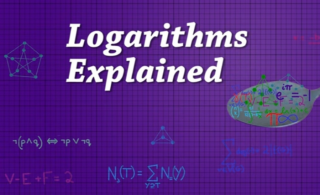Logarithms Explained ▷▷▷
In the same way that division is the inverse of multiplication a logarithm is just the inverse of exponentiation.
3738

Logarithms Explained
What is the power that I should raise this base to in order to get this number?
9635

Logarithms Explained
Anytime you see log base b of some number n, you can think about it as asking the question "What power do I need to put in my exponent to get b to that power equal to this number n?"
9637Saying Logarithm
By definition, when we say log base b of x equals y, that's the same thing as saying "b to the y equals x". So if you want to find log base b of x, you're asking "what power do you have to raise b to in oder to get x?"
3795-